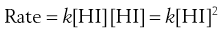# Kinetics

Filed under Basic Science

rate 1 star rate 2 star rate 3 star rate 4 star rate 5 star# Kinetics

W. John Pugh

Chapter contents

## Introduction

Kinetics is the study of the rate at which processes occur. The changes may be chemical (e.g. decomposition of a drug, radiochemical decay) or physical (e.g. transfer across a boundary such as the intestinal lining or the skin). Kinetic studies are useful in providing information that:

In general, the theories and laws of chemical kinetics are well founded and provide a sound basis for the application of such studies to pharmaceutical problems that involve chemical and physical reactions.

The kinetics of processes have importance in a large number of areas of product design, manufacture, storage and drug delivery. These include: dissolution processes (Chapter 2), microbiological growth and destruction (Part 3), biopharmaceutics, including drug absorption, distribution, metabolism and excretion (Part 4), preformulation (Chapter 23), the rate of drug release from dosage forms (Part 5), and the decomposition of medicinal compounds and products (Part 6).

## Homogeneous and heterogeneous reactions

Homogeneous reactions occur in a single phase, i.e. true solutions or gases, and proceed uniformly throughout the whole of the system. Heterogeneous reactions involve more than one phase and are often confined to the phase boundary, their rates being dependent on the supply of fresh reactants to this boundary. Examples are decomposition of drugs in suspensions and enzyme-catalysed reactions.

## Molecularity

Molecularity is the number of molecules involved in forming the product. This follows from the balanced (stoichiometrical) equation describing the reaction. For example, in the following two-step reaction: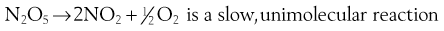and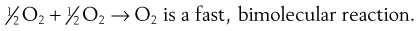## Order

This is the number of concentration terms that determine the rate. In a unimolecular process a molecule will react if it has sufficiently high energy. The number of high-energy molecules depends on how many molecules are present, i.e. on their concentration in solution (or pressure in a gas). In a bimolecular process, two molecules must collide to react and the likelihood of collision depends on the concentrations of each species. The Law of Mass Action states that the rate depends on the product of concentrations of the reactants.

Thus in the first step of the example reaction: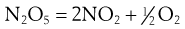the rate of reaction = k1[N2O5]. Here k1 is the rate constant. The square brackets here, and throughout this chapter, mean ‘concentration of the entity within the brackets’. In this case there is only one concentration term and the reaction is known as first order.

In the second step: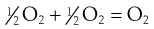the rate of reaction =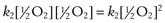, where k2 is the reaction rate constant. Thus there are two concentration terms and the reaction is known as second order.

Each of these is discussed in more detail below.

### First-order processes

The rate is determined by one concentration term. These are by far the most important processes in pharmaceutical science. Many drug decompositions during storage and the passage of drugs from one body compartment to another, e.g. lumen of the intestine into blood, follow first-order kinetics. The rate of reaction is most simply defined as the concentration change divided by the corresponding time change.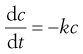(7.1)

The negative sign is used because concentration falls as time increases. This makes the rate constant, k, positive.

The differential equation above describes infinitely small changes. For real changes, these small changes are summed (integrated) usually from the start of the process (time = 0, concentration = co) to the concentration, c, remaining at any other time, t, as follows.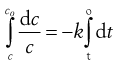(7.2)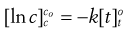(7.3)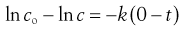(7.4)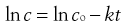(7.5)

Thus a plot of ln c against t is a straight line with intercept ln c0 and gradient −k (Fig. 7.1).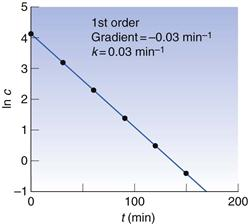The units of k are given by rearranging Equation 7.1, i.e.: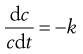(7.6)

Thus the rate constant has the dimensions of time−1 and typical units of s−1.

Note that because k contains no concentration term, it is not necessary to convert experimental data to concentration values in order to estimate it. Any convenient property of the system which is directly proportional to concentration can be used, such as UV absorbance, conductivity, pressure and radioactivity. For example, absorbance (A) is related to concentration, c, by Beer’s Law, i.e. c = αA, where α is a proportionality constant.

Substitution into Equation 7.5 gives: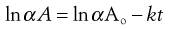(7.7)

hence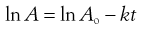(7.8)

Thus the gradient is unchanged from Equation 7.7.

Box 7.1 Worked example

Consider the following example. A radio-labelled cardiac stimulant is administered by intravenous injection. Blood samples have the following radioactivity counts per second (cps).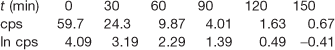From Figure 7.1, it can be seen that the rate constant for absorption from the blood is 0.03 min−1.

### Pseudo first-order processes

Consider the hydrolysis of ethyl acetate: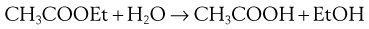Strictly, the reaction is second order and the rate of reaction is expressed as follows. Again the square brackets used here and elsewhere in this chapter imply ‘concentration of’.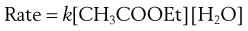However, in a dilute aqueous solution of ethyl acetate, [H2O] is very large compared to [CH3COOEt] and hardly alters during the course of the reaction. [H2O] can be taken as a constant and incorporated into the second-order rate constant, now k′, where k′ = k[H2O]: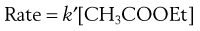Thus, the reaction is, in effect, first order with a rate constant k′. This applies to many drug decompositions by hydrolysis in aqueous solution.

### Second-order processes

The rate depends on the product of two concentration terms. In the simplest case they refer to the same species. For example: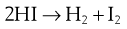Here the reaction is not simply a matter of a HI molecule falling apart, but relies on the collision of two HI molecules. The rate of reaction for this example is given from the Law of Mass Action by: